On of the more interesting qualities of an object is that an object can contain a reference to another object of the same type. There is a common data structure, the linked list, that takes advantage of this feature.

Linked lists are made up of nodes, where each node contains a pointer to the next node in the list. In addition, each node contains a unit of data called the cargo. In our first example, the cargo will be a single integer, but later we will write a generic data structure that can contain objects of any type.

## 16.1. Revenge of the `Node`¶

As usual when we write a new class, we’ll start with the instance variables, one or two constructors and `to_str` so that we can test the basic mechanisms of creating and displaying the new type.

```struct Node {
int cargo;
Node* next;

Node() {
cargo = 0;
next = NULL;
}

Node(int cargo, Node* next) {
this->cargo = cargo;
this->next = next;
}

string to_str() const {
}
};
```

The declarations of the instance variables follow naturally from the specification, and the rest follows machanically from the instance variables. The only thing new here is the constant value `NULL`, which is defined in `iostream` and is equivalent to `0`. We should also note that we are intentionally using `struct` instead of `class`, so that our instance variables are public by default (see What is a class?).

To test the implementation so far, we would put something like this in `main`:

```Node* node1 = new Node(1, NULL);
cout << node1->to_str() << endl;
```

The result is simply:

```1
```

To make it interesting, we need a list with more than one node!

```Node* node1 = new Node(1, NULL);
Node* node2 = new Node(2, NULL);
Node* node3 = new Node(3, NULL);
```

This code creates three nodes, but we don’t have a list yet because the nodes are not linked. The state diagram looks like this: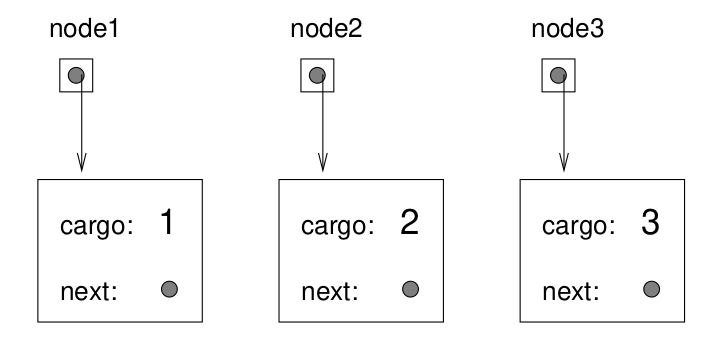To link up the nodes, we have to make the first node refer to the second, and the second node refer to the third.

```node1->next = node2;
node2->next = node3;
```

The `next` variable of `node3` is still `NULL`, which indicates it is the end of the list. Now the state diagram looks like: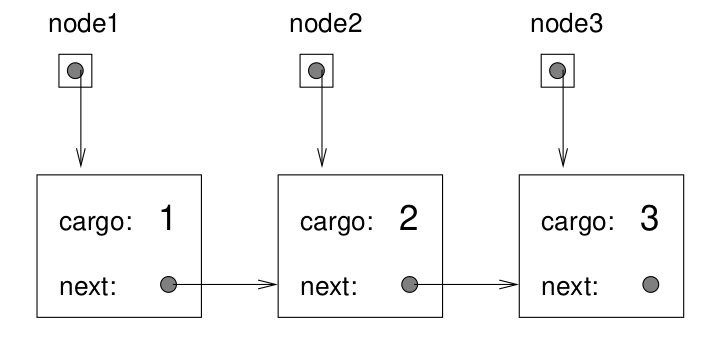Now we know how to create nodes and link them into lists. What might be less clear at this point is why. We’ll take that up in the next section.

## 16.2. Lists as collections¶

The thing that makes lists useful is that they are a way of assembling multiple objects into a single entity, sometimes called a collection. In this example, the first node of the list serves as a pointer to the entire list.

If we want to pass the list as a parameter, all we have to pass is a pointer to the first node, as in the following function:

```void print_list(Node* list) {
Node* node = list;
while (node != NULL) {
cout << node->cargo;
node = node->next;
if (node != NULL) cout << ", ";
}
}
```

We can now call this function, passing it a pointer to the first node in our list:

```print_list(node1);
```

Inside `print_list` we have a pointer to the first node of the list, but there is no variable that refers to the other nodes. We have to use the next value from each node to get to the next node.

This diagram shows the value of `list` and the values that `node` takes on: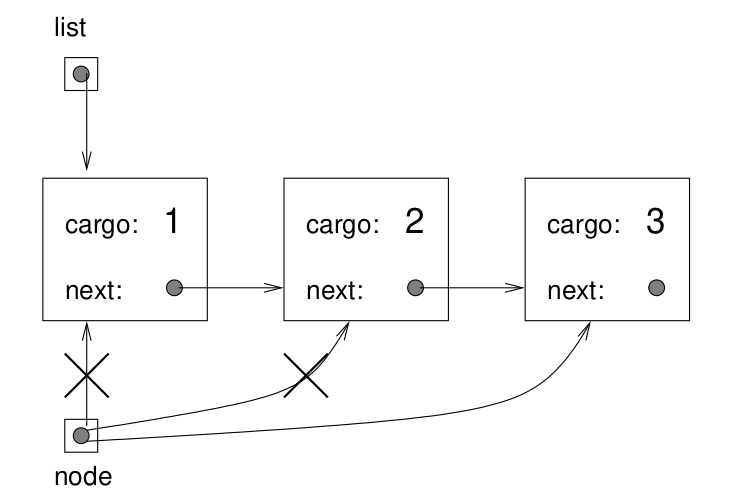This way of moving through a list is called a traversal, just like the similar pattern of moving through the elements of a vector. It is common to use a loop variable like node to point to each of the nodes in the list in succession.

The output of this function is:

```1, 2, 3
```

## 16.3. Lists and recursion¶

Recursion and lists go together like fava beans and a nice Chianti. For example here is a recursive algorithm for printing a list backwards:

1. Separate the list into two pieces: the first node (called the head) and the rest (called the tail).

2. Print the tail backwards.

Of course, step 2, the recursive call, assumes that we have a way of printing a list backwards. But if we assume that the recursive call works – the leap of faith – then we can convince ourselves that this algorithm works.

All we need is a base case, and a way of proving that for any list we will eventually get to the base case. A natural choice for the base case is a list with a single element, but an even better choice is the empty list, represented by `NULL`.

```void print_backward(Node* list) {
if (list == NULL) return;

Node* tail = list->next;

print_backward(tail);
if (head->next != NULL) cout << ", ";
}
```

The first line handles the base case by doing nothing. The next two lines split the list into head and tail. The last three lines print the list. Take a minute to think about why we have the `if` statement that prints `", "` before the line that prints `cargo`. If you understand this, you are well on your way to developing your recursive intution.

We call this function exactly as we did `print_list`:

```print_backward(node1);
```

The result is a backward list:

```3, 2, 1
```

Can we prove that this function will always terminate? In other words, will it always reach the base case? In fact, the answer is no. There are some lists that will make this function crash.

## 16.4. Infinite lists¶

There is nothing to prevent a node from pointing back to an earlier node in the list, including itself. For example, this figure shows a list with two nodes, one of which points to itself.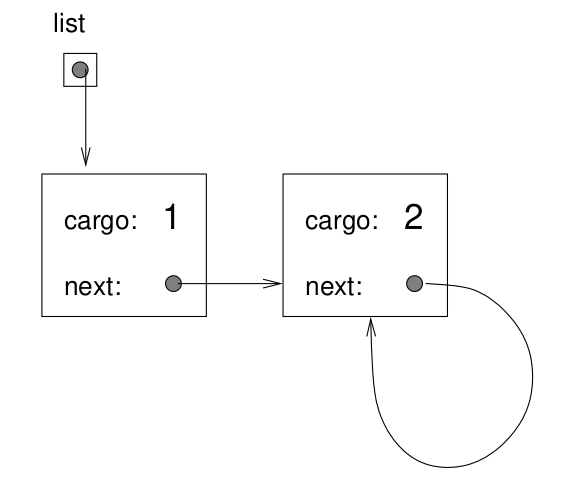If we call either `print_list` with this list as an argument, it will loop forever. If we call `print_backward` it will recurse infinitely. This sort of behavior makes infinite lists difficult to work with.

Nevertheless, they are sometimes very useful. For example, we might represent a number as a list of digits and use an infinite list to represent a repeating fraction.

Regardless, it is problematic that we cannot prove that `print_list` and `print_backward` terminate. The best we can do is the hypothetical statement, “If the list contains no loops, then these functions will terminate.” This is another example of a precondition, to which we were introduced in the Preconditions section of an early chapter. As with the earlier example, it imposes a constraint on one of the parameters and describes the behavior of the function if the constraint is satisfied. We will see more examples of this soon.

## 16.5. The fundamental ambiguity theorem¶

There is a part of `print_backward` that might have raised an eyebrow:

```Node* head = list;
Node* tail = list->next;
```

After the first assignment, `head` and `list` have the same type and the same value. So why did we create a new variable?

The reason is that the two variables play different roles. We think of `head` as a pointer to a single node, and we think of `list` as a pointer to the whole list. These “roles” are not part of the program; they are in the mind of the programmer.

This ambiguity is useful, but it can make programs with lists difficult to read. It is a good practice to use variable names like `node` and `list` to communicate how we intend to use them. Such self-documenting code is easier to read and easier to maintain.

We could have written `print_backward` without `head` and `tail`, but it makes it harder to understand:

```void print_backward(Node* list) {
if (list == NULL) return;

print_backward(list->next);
cout << list->cargo;
}
```

Looking at the two function calls, we have to remember that `print_backward` treats its argument as a list and `cout` treats it as a single node.

Always keep in mind the fundamental ambiguity theorem:

A variable that points to a node might treat the node as a single object or as the first in a list of nodes.

## 16.6. Member functions for nodes¶

You might have wondered why we didn’t write `print_list` and `print_backward` as member functions of `Node`. We said back in Objects and functions that any free-standing function taking an object as an argument could also be written as a member function of that object. It’s just a question of which form is cleaner.

In this case there is a legitimate reason to choose free-standing functions. It is legal to send a `NULL` as an argument to a free-standing function, but it is not legal to invoke a member function on a `NULL` object.

```Node* node = NULL;
print_list(node);    // legal
node.print_list();   // WRONG! - Segmentation fault (core dumped)
```

This limitation makes it awkward to write list-manipulating code in a clean, object-oriented style. A little later we will see a way to get around this.

One obvious way to modify a linked list is to change the cargo of one of its nodes, but the more interesting operations are the ones that add, remove or reorder the nodes.

As an example, we’ll write a function that removes the second node in the list and returns a pointer to it.

```Node* remove_second(Node* list) {
Node* first = list;
Node* second = list->next;

// make the first node point to the third
first->next = second->next;

// remove the second node from the list and return a pointer to it
second->next = NULL;
return second;
}
```

We are again using temporary variables to make the code more readable.

Note

Here be dragons!

Care must be taken here not to leak memory, as we discussed in the Dynamic memory allocation and memory leaks section. If the return value from this function is not assigned to a variable by the caller, the memory used for the second node will be leaked.

Adding these lines to `main` will enable us to test it:

```print_list(node1);
Node* second_node = remove_second(node1);
print_list(second_node);
print_list(node1);
cout << endl;
```

Giving us the output:

```1, 2, 3
2
1, 3
```

Here is a state diagram showing the effect of this operation.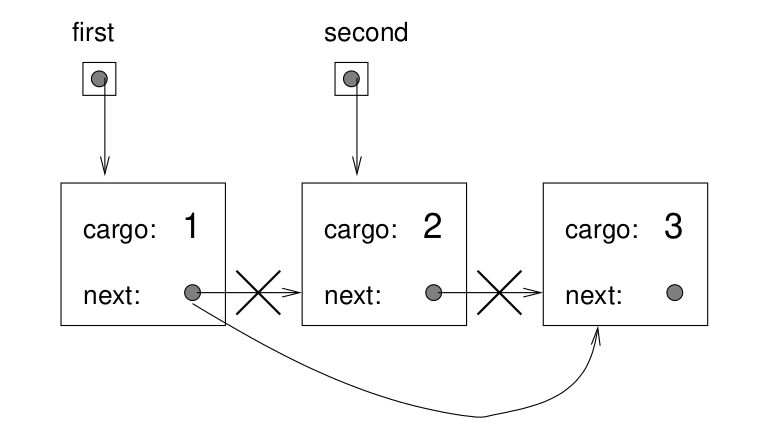What happens if we call this function with a list with only one element (a singleton)? What happens if we pass it an empty list? Should there be a precondition for this function? You’ll be asked to handle these two cases as an exercise.

## 16.8. Wrappers, helpers, and function pointers¶

For some operations it is useful to divide the labor into two functions. For example, we can print a linked list forward with `print_list`, and backward with `print_backward`, and in each case we may want to “pretty print” the list in the conventional format, enclosed in parenthesis, giving us `(1, 2, 3)` and `(3, 2, 1)`.

One of the principles of good software design is the don’t repeat yourself (DRY) principle. Rather than implementing this pretty printing twice, let’s create a wrapper function named `pretty_print` that has a list and a function pointer as parameters.

```void pretty_print(Node* list, void (*list_printer)(Node*)) {
cout << "(";
list_printer(list);
cout << ")" << endl;
}
```

The syntax for creating a pointer to a function is:

```RETURN_TYPE (*FUNCTION_POINTER_VARIABLE_NAME)(FUNCTION_PARAMETERS)
```

This wrapper function is an example of what is called a helper function, since breaks down the parts of a computation (in this case, printing parenthesis and printing the list) into separate functions which can be reused.

We can now call `pretty_print` with the addresses of the functions for printing forward and backward as arguments.

```pretty_print(node1, &print_list);
pretty_print(node1, &print_backward);
```

When we run this we get:

```(1, 2, 3)
(3, 2, 1)
```

There are a number of subtle problems with the way we have been implementing linked lists. The biggest one is the problem we encountered in Member functions for nodes when we considered adding the print functions as member functions to `Node`.

We can solve this problem by wrapping `Node`s in a `LinkedList` class.

```class LinkedList
{
public:
int num_nodes;

num_nodes = 0;
}
}
```

The `LinkedList` class gives us a natural place to put member functions, like the following, that adds a new `Node` to the front of the list:

```void LinkedList::insert_at_front(int cargo) {
Node* front = new Node(cargo, head);
num_nodes++;
}
```

You will be asked to develop this `LinkedList` class further in the exercises, but first we want to generalize it so that it can be used with different types of data.

## 16.10. Templates¶

One drawback of our `LinkedList` implementation is that the data (stored in the `cargo` instance variable of `Node`s) is limited to a single type, `int` in our present case, even though it probably occurred to you that we might want linked lists with various types of data.

While we could implement a different linked list class for each data type, this would be tedious, and it would violate the DRY principle we discussed earlier.

Fortunately, C++ provides class templates, which give us a solution to this problem.

Templates provide a way to specify linked lists as a generic data structure, meaning they can hold a variety of data types. Using templates on our `Node` class looks like this:

```template <class T>
class Node
{
public:
T cargo;
Node<T>* next;

Node(T cargo, Node<T>* next) {
this->cargo = cargo;
this->next = next;
}
};
```

We begin with a new keyword, `template`, followed by `<class T>`, where `T` represents the datatype that will be used to instantiate the templated class. So our instance variable, `cargo` is given datatype `T`, and the `next` pointer is declared to point to a `Node<T>`.

We can repeat the process of creating an explicitely linking together three nodes and than printing out their contents, this time with nodes containing string data, in a file named `test_templated_nodes.cpp`:

```#include <iostream>
#include <string>
using namespace std;

int main()
{
Node<string>* node1 = new Node<string>("I", NULL);
Node<string>* node2 = new Node<string>("love", NULL);
Node<string>* node3 = new Node<string>("templates!", NULL);

node1->next = node2;
node2->next = node3;

Node<string>* node = node1;

while (node != NULL) {
cout << node->cargo << endl;
node = node->next;
}

return 0;
}
```

The next step is to use templating for our new `LinkedList` class.

```template <class T>
{
public:
int num_nodes;

// constructor
num_nodes = 0;
}

// modifiers
void insert_at_front(T cargo) {
Node<T>* front = new Node<T>(cargo, head);
num_nodes++;
}

T remove_from_front() {
throw runtime_error("Can't remove from empty list!");
delete front;
num_nodes--;
return cargo;
}
};
```

The instance variable `head` now points to the templated node, `Node<T>*`, as do the nodes we create in `insert_at_front` and `remove_from_front`. We are careful to free up the released memory in `remove_from_front` using the `delete` operator, as we discussed in Dynamic memory allocation and memory leaks. To do this we need to make use of a temporary variable, `front`. It points to the old head after we advance head to next, so we can then delete it.

We also have to decide what to do when `remove_from_front` is called on an empty list. For now, we are using the C++ throw command to report an error. This will cause the program to halt, so client code should take pains to avoid calling `remove_from_front` on an empty list. We’ll discuss this further in the Exceptions section next chapter.

A more complete listing of code for this class is given in Appendix A: Code Source, including another constructor, and member functions to return its length and render it as a string. You will be asked to add several additional member functions to it in the exercises.

## 16.11. Invariants¶

Some linked lists are “well-formed,” others are not. For example, if a list contains a loop, it will cause many of our functions to crash, so we might want to require that lists contain no loops. Another requirement is that the `num_nodes` value in a `LinkedList` object should be equal to the actual number of nodes in the list.

Requirements like these are called invariants because, ideally, they should be true of every object all the time. Specifying invariants for objects is a useful programming practice because it makes it easier to prove the correctness of code, check the integrity of data structures, and detect errors.

One thing that is sometimes confusing about invariants is that there are some times when they are violated. For example, in the middle of `insert_at_front`, after we have added the node, but before we have incremented `num_nodes`, the invariant is violated. This kind of violation is acceptable; in fact, it is often impossible to modify an object without violating an invariant for at least a little while. Normally the requirement is that every function that violates an invariant must restore the invariant before it finishes.

## 16.12. Glossary¶

A data structure that implements a collection using a sequence of linked nodes.

node

An element of a list, usually implemented as an object that contains a pointer to another object of the same type.

cargo

An item of data contained in a node.

A pointer to a node embedded in a node.

generic data structure

A kind of data structure that can contain data of any type.